LR  >  Mirror Images Quiz I, Non Verbal Reasoning

# Mirror Images Quiz I, Non Verbal Reasoning

Test Description

## 25 Questions MCQ Test General Aptitude for GATE | Mirror Images Quiz I, Non Verbal Reasoning

Mirror Images Quiz I, Non Verbal Reasoning for LR 2023 is part of General Aptitude for GATE preparation. The Mirror Images Quiz I, Non Verbal Reasoning questions and answers have been prepared according to the LR exam syllabus.The Mirror Images Quiz I, Non Verbal Reasoning MCQs are made for LR 2023 Exam. Find important definitions, questions, notes, meanings, examples, exercises, MCQs and online tests for Mirror Images Quiz I, Non Verbal Reasoning below.
Solutions of Mirror Images Quiz I, Non Verbal Reasoning questions in English are available as part of our General Aptitude for GATE for LR & Mirror Images Quiz I, Non Verbal Reasoning solutions in Hindi for General Aptitude for GATE course. Download more important topics, notes, lectures and mock test series for LR Exam by signing up for free. Attempt Mirror Images Quiz I, Non Verbal Reasoning | 25 questions in 50 minutes | Mock test for LR preparation | Free important questions MCQ to study General Aptitude for GATE for LR Exam | Download free PDF with solutions
 1 Crore+ students have signed up on EduRev. Have you?
Mirror Images Quiz I, Non Verbal Reasoning - Question 1

### Directions to Solve In each of the following questions you are given a combination of alphabets and/or numbers followed by four alternatives (1), (2), (3) and (4). Choose the alternative which is closely resembles the mirror image of the given combination. Question - Choose the alternative which is closely resembles the mirror image of the given combination.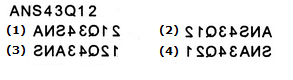Mirror Images Quiz I, Non Verbal Reasoning - Question 2

### Directions to Solve In each of the following questions you are given a combination of alphabets and/or numbers followed by four alternatives (1), (2), (3) and (4). Choose the alternative which is closely resembles the mirror image of the given combination. Question - Choose the alternative which is closely resembles the mirror image of the given combination.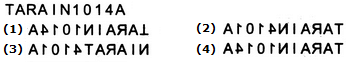Mirror Images Quiz I, Non Verbal Reasoning - Question 3

### Directions to Solve In each of the following questions you are given a combination of alphabets and/or numbers followed by four alternatives (1), (2), (3) and (4). Choose the alternative which is closely resembles the mirror image of the given combination. Question - Choose the alternative which is closely resembles the mirror image of the given combination.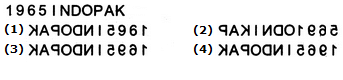Mirror Images Quiz I, Non Verbal Reasoning - Question 4

In each of the following questions you are given a combination of alphabets and/or numbers followed by four alternatives (1), (2), (3) and (4). Choose the alternative which is closely resembles the mirror image of the given combination.

Question -

Choose the alternative which is closely resembles the mirror image of the given combination.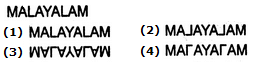Mirror Images Quiz I, Non Verbal Reasoning - Question 5

5.Choose the alternative which is closely resembles the mirror image of the given combination.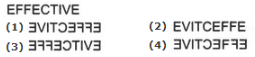Mirror Images Quiz I, Non Verbal Reasoning - Question 6

In each of the following questions you are given a combination of alphabets and/or numbers followed by four alternatives (1), (2), (3) and (4). Choose the alternative which is closely resembles the mirror image of the given combination.

Question -

Choose the alternative which is closely resembles the mirror image of the given combination.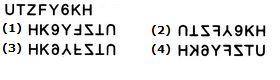Mirror Images Quiz I, Non Verbal Reasoning - Question 7

In each of the following questions you are given a combination of alphabets and/or numbers followed by four alternatives (1), (2), (3) and (4). Choose the alternative which is closely resembles the mirror image of the given combination.

Question -

Choose the alternative which is closely resembles the mirror image of the given combination.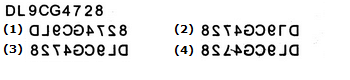Mirror Images Quiz I, Non Verbal Reasoning - Question 8

Directions to Solve

In each of the following questions you are given a combination of alphabets and/or numbers followed by four alternatives (1), (2), (3) and (4). Choose the alternative which is closely resembles the mirror image of the given combination.

Question -

Choose the alternative which is closely resembles the mirror image of the given combination.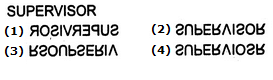Detailed Solution for Mirror Images Quiz I, Non Verbal Reasoning - Question 8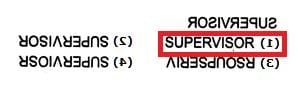Mirror Images Quiz I, Non Verbal Reasoning - Question 9

In each of the following questions you are given a combination of alphabets and/or numbers followed by four alternatives (1), (2), (3) and (4). Choose the alternative which is closely resembles the mirror image of the given combination.

Question -

Choose the alternative which is closely resembles the mirror image of the given combination.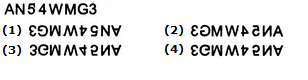Mirror Images Quiz I, Non Verbal Reasoning - Question 10

In each of the following questions you are given a combination of alphabets and/or numbers followed by four alternatives (1), (2), (3) and (4). Choose the alternative which is closely resembles the mirror image of the given combination.

Question -

Choose the alternative which is closely resembles the mirror image of the given combination.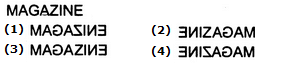Mirror Images Quiz I, Non Verbal Reasoning - Question 11

In each of the following questions you are given a combination of alphabets and/or numbers followed by four alternatives (1), (2), (3) and (4). Choose the alternative which is closely resembles the mirror image of the given combination.

Question -

Choose the alternative which is closely resembles the mirror image of the given combination.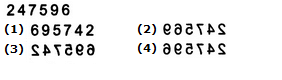Mirror Images Quiz I, Non Verbal Reasoning - Question 12

In each of the following questions you are given a combination of alphabets and/or numbers followed by four alternatives (1), (2), (3) and (4). Choose the alternative which is closely resembles the mirror image of the given combination.

Question -

Choose the alternative which is closely resembles the mirror image of the given combination.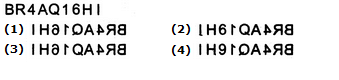Mirror Images Quiz I, Non Verbal Reasoning - Question 13

In each of the following questions you are given a combination of alphabets and/or numbers followed by four alternatives (1), (2), (3) and (4). Choose the alternative which is closely resembles the mirror image of the given combination.

Question -

Choose the alternative which is closely resembles the mirror image of the given combination.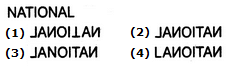Mirror Images Quiz I, Non Verbal Reasoning - Question 14

In each of the following questions you are given a combination of alphabets and/or numbers followed by four alternatives (1), (2), (3) and (4). Choose the alternative which is closely resembles the mirror image of the given combination.

Question -

Choose the alternative which is closely resembles the mirror image of the given combination.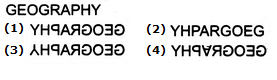Mirror Images Quiz I, Non Verbal Reasoning - Question 15

In each of the following questions you are given a combination of alphabets and/or numbers followed by four alternatives (1), (2), (3) and (4). Choose the alternative which is closely resembles the mirror image of the given combination.

Question -

Choose the alternative which is closely resembles the mirror image of the given combination.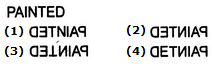Mirror Images Quiz I, Non Verbal Reasoning - Question 16

In each of the following questions you are given a combination of alphabets and/or numbers followed by four alternatives (1), (2), (3) and (4). Choose the alternative which is closely resembles the mirror image of the given combination.

Question -

Choose the alternative which is closely resembles the mirror image of the given combination.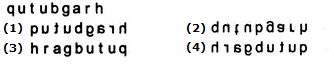Mirror Images Quiz I, Non Verbal Reasoning - Question 17

In each of the following questions you are given a combination of alphabets and/or numbers followed by four alternatives (1), (2), (3) and (4). Choose the alternative which is closely resembles the mirror image of the given combination.

Question -

Choose the alternative which is closely resembles the mirror image of the given combination.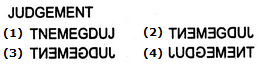Mirror Images Quiz I, Non Verbal Reasoning - Question 18

In each of the following questions you are given a combination of alphabets and/or numbers followed by four alternatives (1), (2), (3) and (4). Choose the alternative which is closely resembles the mirror image of the given combination.

Question -

Choose the alternative which is closely resembles the mirror image of the given combination.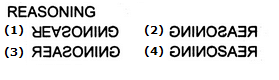Mirror Images Quiz I, Non Verbal Reasoning - Question 19

In each of the following questions you are given a combination of alphabets and/or numbers followed by four alternatives (1), (2), (3) and (4). Choose the alternative which is closely resembles the mirror image of the given combination.

Question -

Choose the alternative which is closely resembles the mirror image of the given combination.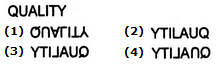Mirror Images Quiz I, Non Verbal Reasoning - Question 20

Directions to Solve

In each of the following questions you are given a combination of alphabets and/or numbers followed by four alternatives (1), (2), (3) and (4). Choose the alternative which is closely resembles the mirror image of the given combination.

Question -

Choose the alternative which is closely resembles the mirror image of the given combination.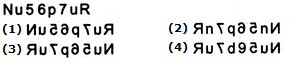Detailed Solution for Mirror Images Quiz I, Non Verbal Reasoning - Question 20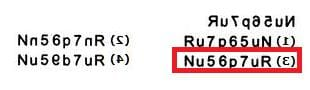Mirror Images Quiz I, Non Verbal Reasoning - Question 21

Directions to Solve

In each of the following questions you are given a combination of alphabets and/or numbers followed by four alternatives (1), (2), (3) and (4). Choose the alternative which is closely resembles the mirror image of the given combination.

Question -

Choose the alternative which is closely resembles the mirror image of the given combination.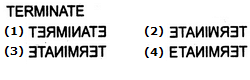Detailed Solution for Mirror Images Quiz I, Non Verbal Reasoning - Question 21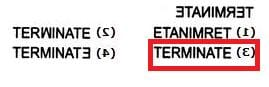Mirror Images Quiz I, Non Verbal Reasoning - Question 22

In each of the following questions you are given a combination of alphabets and/or numbers followed by four alternatives (1), (2), (3) and (4). Choose the alternative which is closely resembles the mirror image of the given combination.

Question -

Choose the alternative which is closely resembles the mirror image of the given combination.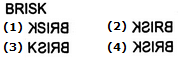Mirror Images Quiz I, Non Verbal Reasoning - Question 23

Directions to Solve

In each of the following questions you are given a combination of alphabets and/or numbers followed by four alternatives (1), (2), (3) and (4). Choose the alternative which is closely resembles the mirror image of the given combination.

Question -

Choose the alternative which is closely resembles the mirror image of the given combination.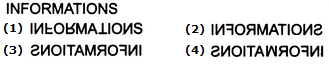Detailed Solution for Mirror Images Quiz I, Non Verbal Reasoning - Question 23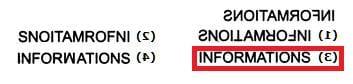Mirror Images Quiz I, Non Verbal Reasoning - Question 24

In each of the following questions you are given a combination of alphabets and/or numbers followed by four alternatives (1), (2), (3) and (4). Choose the alternative which is closely resembles the mirror image of the given combination.

Question -

Choose the alternative which is closely resembles the mirror image of the given combination.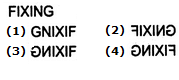Mirror Images Quiz I, Non Verbal Reasoning - Question 25

In each of the following questions you are given a combination of alphabets and/or numbers followed by four alternatives (1), (2), (3) and (4). Choose the alternative which is closely resembles the mirror image of the given combination.

Question -

Choose the alternative which is closely resembles the mirror image of the given combination.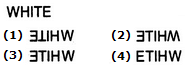## General Aptitude for GATE

73 videos|86 docs|108 tests
 Use Code STAYHOME200 and get INR 200 additional OFF Use Coupon Code
Information about Mirror Images Quiz I, Non Verbal Reasoning Page
In this test you can find the Exam questions for Mirror Images Quiz I, Non Verbal Reasoning solved & explained in the simplest way possible. Besides giving Questions and answers for Mirror Images Quiz I, Non Verbal Reasoning, EduRev gives you an ample number of Online tests for practice

## General Aptitude for GATE

73 videos|86 docs|108 tests